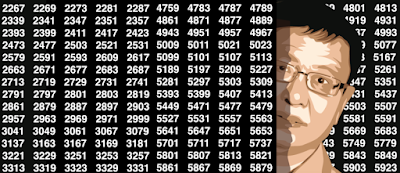## Friday, June 23, 2017

### 12 Interesting Reads for Math Geeks

Many data scientists have a passion for mathematics, and many modern math problems can be explored using data science. Below is a selection of interesting articles, many about challenging, deep mathematical problems, by a data scientist who developed math-free algorithms. Some of these articles cover statistical theory and thus belong to data science, some are just about mathematics and number theory for its own sake. Most of them can be understood by the layman. Some include R code to produce visualizations, and some include processing vast amounts of data -- trillions of data points: thus it provides an excellent sandbox to test distributed architecture implementations, and high performance computing.
12 Interesting Reads for Math Geeks
DSC Resources